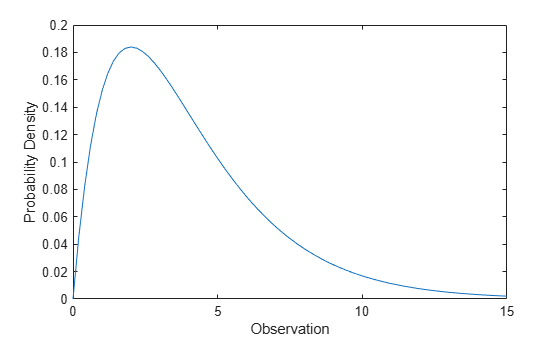Documentation

This is machine translation

Mouseover text to see original. Click the button below to return to the English version of the page.

Chi-Square Distribution

Overview

The chi-square distribution is commonly used in hypothesis testing, particularly the chi-squared test for goodness of fit.

Parameters

The chi-square distribution uses the following parameter.

ParameterDescriptionSupport
νDegrees of freedomν is a positive value

Probability Density Function

The probability density function (pdf) is

`$y=f\left(x|\nu \right)=\frac{{x}^{\left(\nu -2\right)/2}{e}^{-x/2}}{{2}^{\frac{\nu }{2}}\Gamma \left(\nu /2\right)}$`

where Γ( · ) is the Gamma function, ν is the degrees of freedom, and x`0`.

Cumulative Distribution Function

The cumulative distribution function (cdf) is

`$p=F\left(x|\nu \right)={\int }_{0}^{x}\frac{{t}^{\left(\nu -2\right)/2}{e}^{-t/2}}{{2}^{\nu /2}\Gamma \left(\nu /2\right)}dt$`

where Γ( · ) is the Gamma function, ν is the degrees of freedom, and x`0`.

Descriptive Statistics

The mean is ν.

The variance is `2`ν.

Relationship to Other Distributions

The χ2 distribution is a special case of the gamma distribution where b = 2 in the equation for gamma distribution below.

`$y=f\left(x|a,b\right)=\frac{1}{{b}^{a}\Gamma \left(a\right)}{x}^{a-1}{e}^{\frac{x}{b}}$`

The χ2 distribution gets special attention because of its importance in normal sampling theory. If a set of n observations is normally distributed with variance σ2, and s2 is the sample variance, then

`$\frac{\left(n-1\right){s}^{2}}{{\sigma }^{2}}\sim {\chi }^{2}\left(n-1\right)$`

This relationship is used to calculate confidence intervals for the estimate of the normal parameter σ2 in the function `normfit`.

Examples

Compute Chi-Square Distribution pdf

Compute the pdf of a chi-square distribution with 4 degrees of freedom.

```x = 0:0.2:15; y = chi2pdf(x,4);```

Plot the pdf.

```figure; plot(x,y)```The chi-square distribution is skewed to the right, especially for few degrees of freedom.